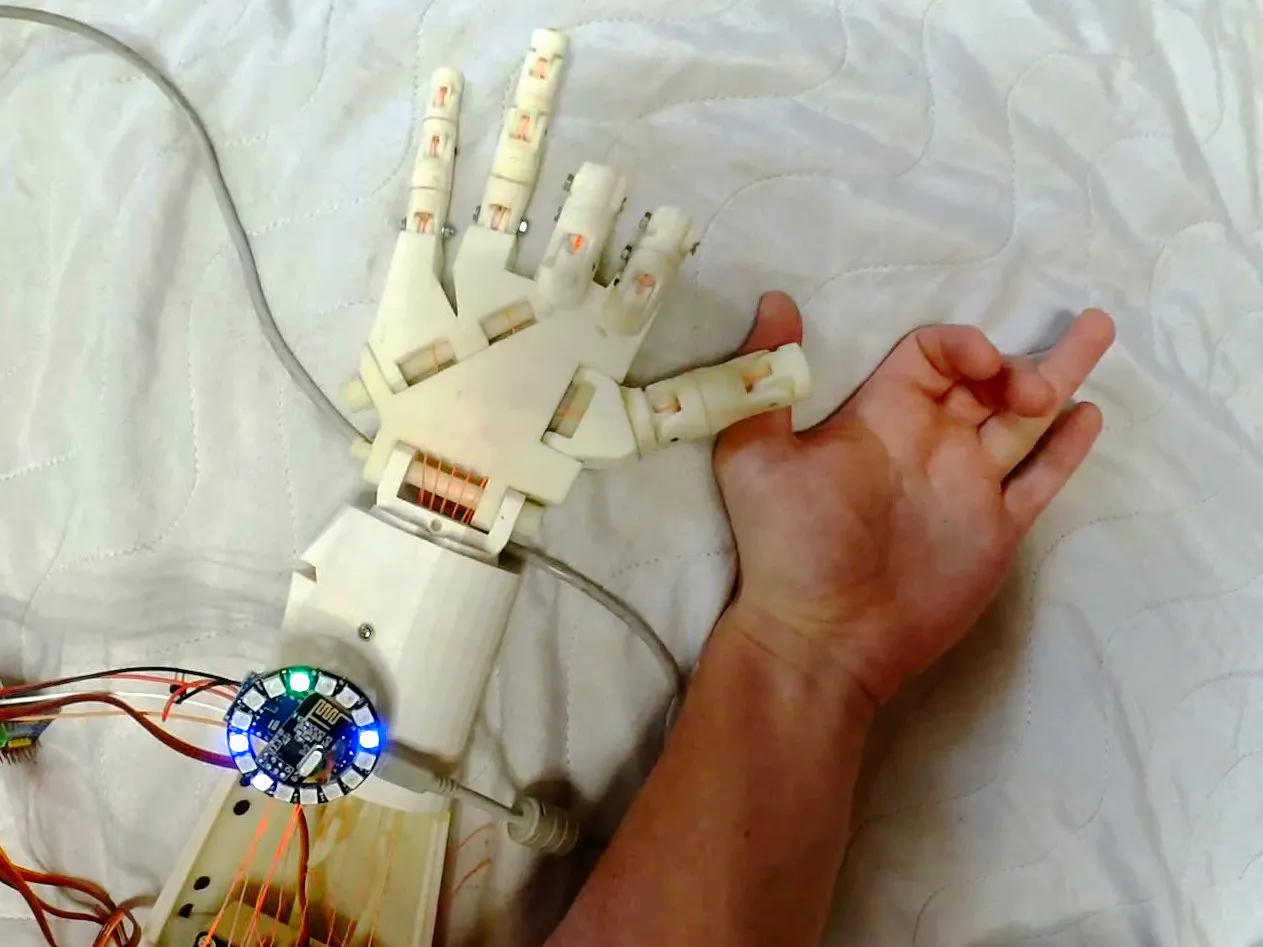# Robotic Hand Control Using EMG

3-channel EMG allows to control individual fingers with very little delay.

ExpertFull instructions providedOver 1 day13,484## Things used in this project

### Hardware components

 uECG device
×3
 inMoov hand
×1Arduino Nano R3
×1
×1
 nRF24 Module (Generic)
×1

## Code

### emg_hand_control2.ino

Arduino
```#include <SPI.h>
#include <RF24.h>
#include <RF24_config.h>
#include <nRF24L01.h>

#include <Wire.h>

#define SERVOMIN  150 // this is the 'minimum' pulse length count (out of 4096)
#define SERVOMAX  600 // this is the 'maximum' pulse length count (out of 4096)

int rf_cen = 9; //nRF24 chip enable pin
int rf_cs = 8; //nRF24 CS pin

RF24 rf(rf_cen, rf_cs);
//pipe address - hardcoded on uECG side
uint8_t pipe_rx = {0x0E, 0xE6, 0x0D, 0xA7, 0, 0, 0, 0};

uint8_t  swapbits(uint8_t a){ //uECG pipe address uses swapped bits order
// reverse the bit order in a single byte
uint8_t v = 0;
if(a & 0x80) v |= 0x01;
if(a & 0x40) v |= 0x02;
if(a & 0x20) v |= 0x04;
if(a & 0x10) v |= 0x08;
if(a & 0x08) v |= 0x10;
if(a & 0x04) v |= 0x20;
if(a & 0x02) v |= 0x40;
if(a & 0x01) v |= 0x80;
return v;
}

long last_servo_upd = 0; //time when we last updated servo values - don't want to do this too often
byte in_pack; //array for incoming RF packet
unsigned long unit_ids = {4294963881, 4294943100, 28358}; //array of known uECG IDs - need to fill with your own unit IDs
int unit_vals = {0, 0, 0}; //array of uECG values with these IDs

float tgt_angles; //target angles for 5 fingers
float cur_angles; //current angles for 5 fingers

float angle_open = 30; //angle that corresponds to open finger
float angle_closed = 150; //angle that corresponds to closed finger

void setup() {
//nRF24 requires relatively slow SPI, probably would work at 2MHz too
SPI.begin();
SPI.setBitOrder(MSBFIRST);
SPI.beginTransaction(SPISettings(1000000, MSBFIRST, SPI_MODE0));

for(int x = 0; x < 8; x++) //nRF24 and uECG have different bit order for pipe address
pipe_rx[x] = swapbits(pipe_rx[x]);

rf.begin();
rf.setDataRate(RF24_1MBPS);
rf.setChannel(22);
rf.setRetries(0, 0);
rf.setAutoAck(0);
rf.setCRCLength(RF24_CRC_DISABLED);
rf.disableCRC();
rf.startListening(); //listen for uECG data
//Note that uECG should be switched into raw data mode (via long button press)
//in order to send compatible packets, by default it sends data in BLE mode
//which cannot be received by nRF24

Serial.begin(115200); //serial output - very useful for debugging

pwm.begin(); //start PWM driver
pwm.setPWMFreq(60);  // Analog servos run at ~60 Hz updates
for(int i = 0; i < 5; i++) //set initial finger positions
{
tgt_angles[i] = angle_open;
cur_angles[i] = angle_open;
}
}

void setAngle(int n, float angle){ //sends out angle value for given channel
pwm.setPWM(n, 0, SERVOMIN + angle * 0.005556 * (SERVOMAX - SERVOMIN));
}

float angle_speed = 15; //how fast fingers would move

float v0 = 0, v1 = 0, v2 = 0; //filtered muscle activity values per 3 channels

void loop()
{
if(rf.available())
{
byte u1 = in_pack;//32-bit unit ID, unique for every uECG device
byte u2 = in_pack;
byte u3 = in_pack;
byte u4 = in_pack;
unsigned long id = (u1<<24) | (u2<<16) | (u3<<8) | u4;
//Serial.println(id); //uncomment this line to make list of your uECG IDs
if(in_pack != 32) id = 0; //wrong pack type: in EMG mode this byte must be 32
int val = in_pack; //muscle activity value
if(val != in_pack) id = 0; //value is duplicated in 2 bytes because RF noise can corrupt packet, and we don't have CRC with nRF24

//find which ID corresponds to current ID and fill value
for(int n = 0; n < 3; n++)
if(id == unit_ids[n])
unit_vals[n] = val;
}
long ms = millis();
if(ms - last_servo_upd > 20) //don't update servos too often
{
last_servo_upd = ms;
for(int n = 0; n < 5; n++) //go through fingers, if target and current angles don't match - adjust them
{
if(cur_angles[n] < tgt_angles[n] - angle_speed/2) cur_angles[n] += angle_speed;
if(cur_angles[n] > tgt_angles[n] + angle_speed/2) cur_angles[n] -= angle_speed;
}
for(int n = 0; n < 5; n++) //apply angles to fingers
setAngle(n, cur_angles[n]);

//exponential averaging: prevents single peaks from affecting finger state
v0 = v0*0.7 + 0.3*(float)unit_vals;
v1 = v1*0.7 + 0.3*(float)unit_vals;
v2 = v2*0.7 + 0.3*(float)unit_vals;

//calcualting scores from raw values
float scor0 = 4.0*v0*v0/((v1*0.3 + 20)*(v2*1.3 + 15));
float scor1 = 4.0*v1*v1/((v0*2.0 + 20)*(v2*2.0 + 20));
float scor2 = 4.0*v2*v2/((v0*1.2 + 20)*(v1*0.5 + 15));

//print scores for debugging
Serial.print(scor0);
Serial.print(' ');
Serial.print(scor1);
Serial.print(' ');
Serial.println(scor2);

//compare each score with threshold and change finger states correspondingly
if(scor2 < 0.5) //weak signal - open finger
tgt_angles = angle_open;
if(scor2 > 1.0) //strong signal - close finger
tgt_angles = angle_closed;

if(scor1 < 0.5)
{
tgt_angles = angle_open;
tgt_angles = angle_open;
}
if(scor1 > 1.0)
{
tgt_angles = angle_closed;
tgt_angles = angle_closed;
}

if(scor0 < 0.5)
{
tgt_angles = angle_open;
tgt_angles = angle_open;
}
if(scor0 > 1.0)
{
tgt_angles = angle_closed;
tgt_angles = angle_closed;
}
}
}
```

## Credits

### Dmitry Dziuba

3 projects • 34 followers
I'm an electronics engineer with background in AI and physics